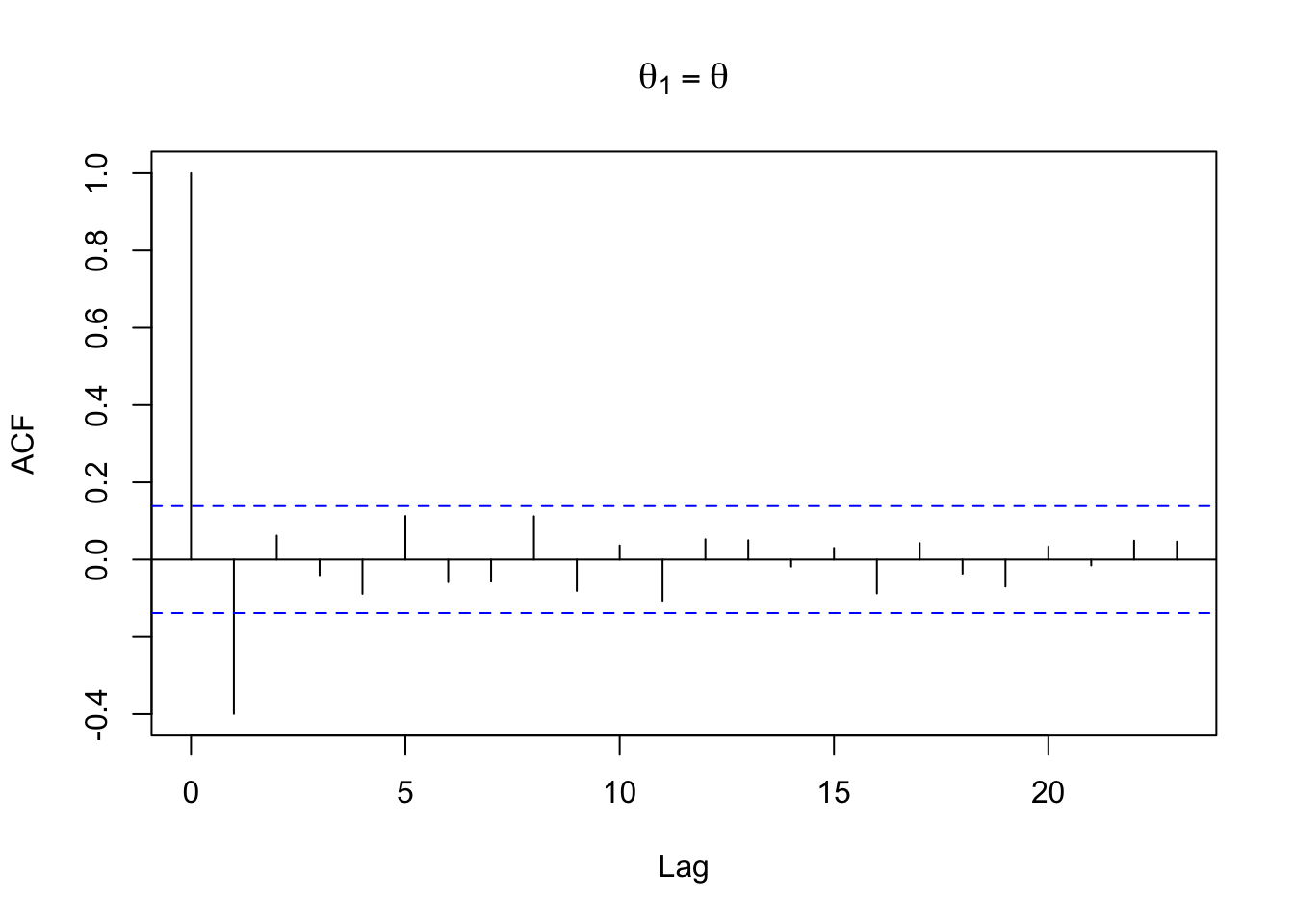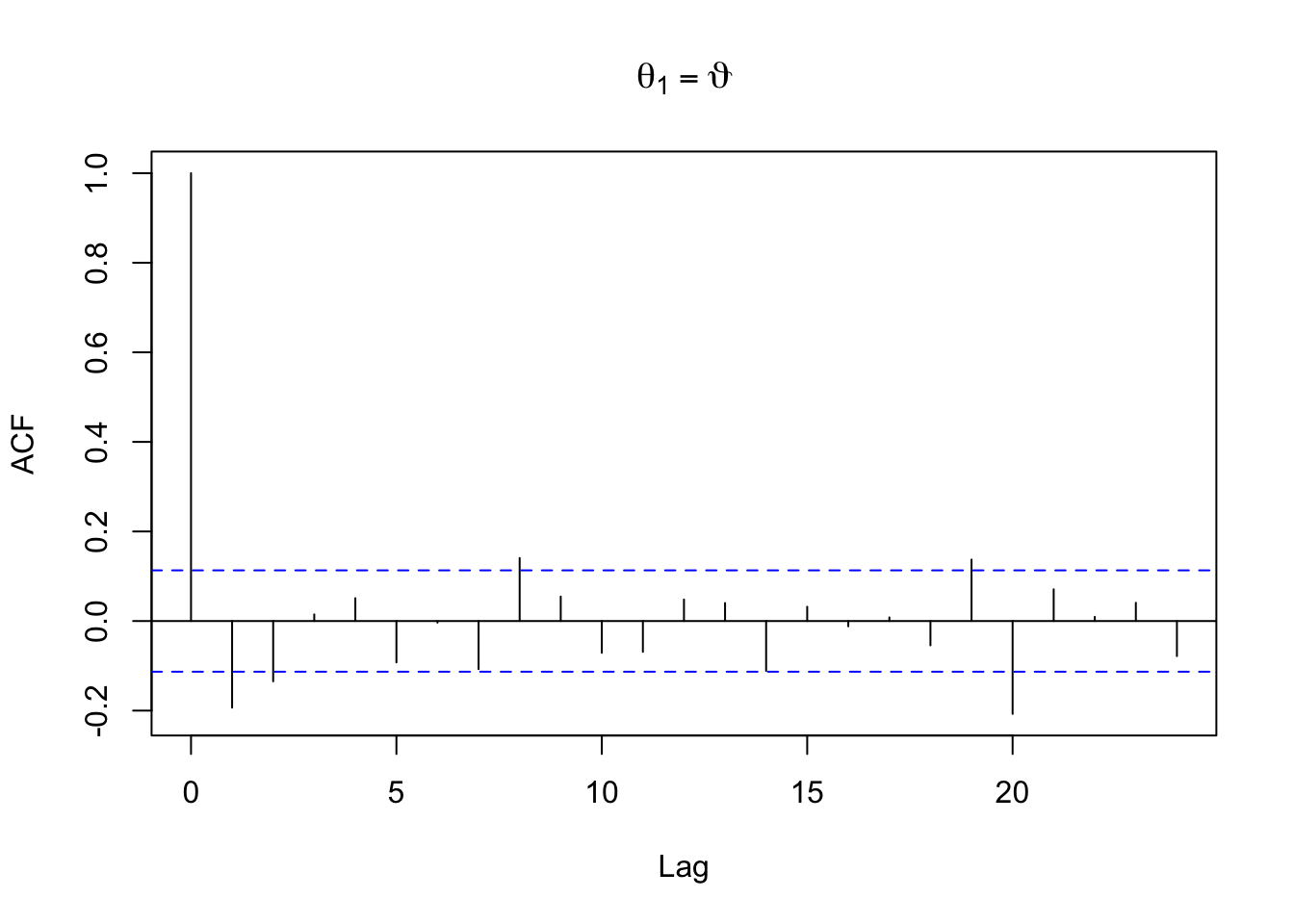#4

set.seed(0)
theta <- -0.5
x <- arima.sim(n = 200,model = list(ma=c(theta)))
y <- acf(x,main = expression(theta == theta))acf1 = y$acf In the ACF plot we can see the different values for the sample autocorrelation $$\rho(.)$$ as a function of the lag between observations. The first value is $$\rho(0)$$ which is equal to 1 by definition. The estimate of $$\rho(1)$$ is −0.399, which is very close to the true theoretical value $$\rho(1)$$ = −0.4 . Taking into the account of an MA(q) process where $$\rho(h)$$ = 0 for all |h| > q, the remaining values of the sample ACF contained between the confidence bound in dotted blue lines are statistically not significantly different from zero. # 5 set.seed(5) theta1 <- 2 theta2 <- -8 x <- arima.sim(n = 300,model = list(ma=c(theta1,theta2))) y <- acf(x,main = expression(theta == theta1, theta == theta))rho2 = y$acf

In the ACF plot we can see the different values for the sample autocorrelation $$\rho(·)$$ as a function of the lag between observations. $$\rho(0)$$ = 1 by definition, $$\rho(1)$$ = −0.193 and $$\rho(2)$$ = −0.135 . The sample estimate for $$\rho(2)$$, -0.135, differs a bit from the true value of $$\rho(2)$$, -0.116.